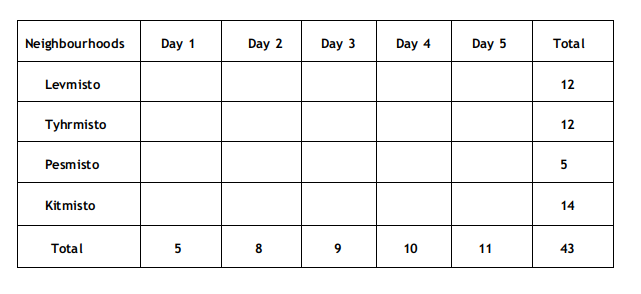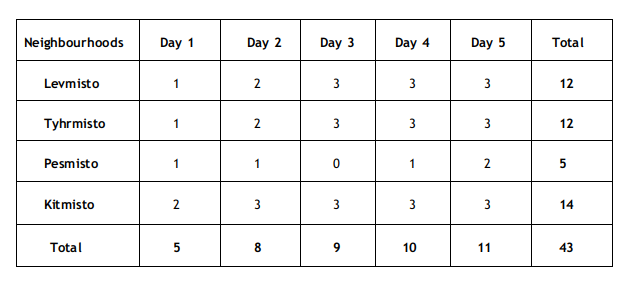### CAT 2022 Question Paper (Slot 3) Question 30

Instructions

There are only four neighbourhoods in a city - Levmisto, Tyhrmisto, Pesmisto and Kitmisto.During the onset of a pandemic, the number of new cases of a disease in each of these neighbourhoods was recorded over a period of five days. On each day, the number of newcases recorded in any of the neighbourhoods was either 0, 1, 2 or 3.

The following facts are also known:

1.There was at least one new case in every neighbourhood on Day 1.
2.On each of the five days, there were more new cases in Kitmisto than in Pesmisto.
3.The number of new cases in the city in a day kept increasing during the five-day period. The number of new cases on Day 3 was exactly one more than that on Day 2.
4.The maximum number of new cases in a day in Pesmisto was 2, and this happened only once during the five-day period.
5.Kitmisto is the only place to have 3 new cases on Day 2.
6.The total numbers of new cases in Levmisto, Tyhrmisto, Pesmisto and Kitmisto over the five-day period were 12, 12, 5 and 14 respectively.

Question 30

# What BEST can be concluded about the total number of new cases in the city on Day2?

Solution

From statement 6, it can be concluded that the total number of new cases is equal to 12+12+5+14 = 43.

Also, since the total number of cases in Kitmisto is 14, it can be concluded that the number of cases each day is either 2 or 3, where 3 cases are recorded on 4 days and 2 cases are recorded on 1 day.

From statement 4, it can be concluded that the number of new cases for Pesmisto will be 0,1,1,1, and 2, in any order(Since the total number of cases is 5, and the maximum number of new cases is 2).

In statement 3, it is given that the number of new cases kept increasing during the 5-day period.

Now, as it is already known that the maximum number of cases for Pesmisto is 2, the maximum total number of cases in a day(or on day 5) will be less than 12.

Let us consider the maximum number of cases on Day 5 as 10.

Thus the maximum number of cases possible for the remaining days will be 9, 8, 7, and 6. So, the total number of maximum cases possible for this case will be 40(less than 43).

Thus, the number of cases on Day 5 will be 11(i.e., b/w 10 and 11)

Now, if the number of cases on Day 4 is 9, the maximum number of cases possible for the remaining days will be 8, 7, and 6.

Thus, the maximum number of cases, in this case, will be 41(less than 43).

So, the number of cases on day 4 will be 10.

Now, if the number of cases on Day 3 is 8, the number of cases on day 2 will be 7, and the maximum possible number of cases on Day 1 will be 6.

Thus, the number of cases, in this case, will be 42(less than 43).

Thus, the number of cases on day 3 will be 9, the number of cases on day 2 will be 8, and the number of cases on day 1 will be 5.Since all the neighbourhood has at least one case on Day 1, the only possible combination will be 1, 1, 1, and 2 for Levmisto, Tyhrmisto, Pesmisto and Kitmisto, respectively.

Now, for the other 4 days, the number of cases in Kitmisto will be 3.

For day 5, the number of cases will be 3, 3, 2, and 3 for Levmisto, Tyhrmisto, Pesmisto and Kitmisto, respectively(since the maximum number of cases in Pesmisto is 2).

And since Pesmisto only has the maximum number of cases on one day, the number of cases on day 4 will be 3, 3, 1, and 3 for Levmisto, Tyhrmisto, Pesmisto and Kitmisto, respectively.

On day 2, since Kitmisto is the only neighbourhood to have 3 cases, the number of cases on day 2 will be 2, 2, 1, and 3 for Levmisto, Tyhrmisto, Pesmisto and Kitmisto, respectively.

Now, on day 3, the number of cases will be 3, 3, 0, and 3 for Levmisto, Tyhrmisto, Pesmisto and Kitmisto, respectively.

Thus, the final table will be as follows:From the data, it can be concluded that the total number of cases on Day 2 is equal to 8.

Thus, the correct option is D.